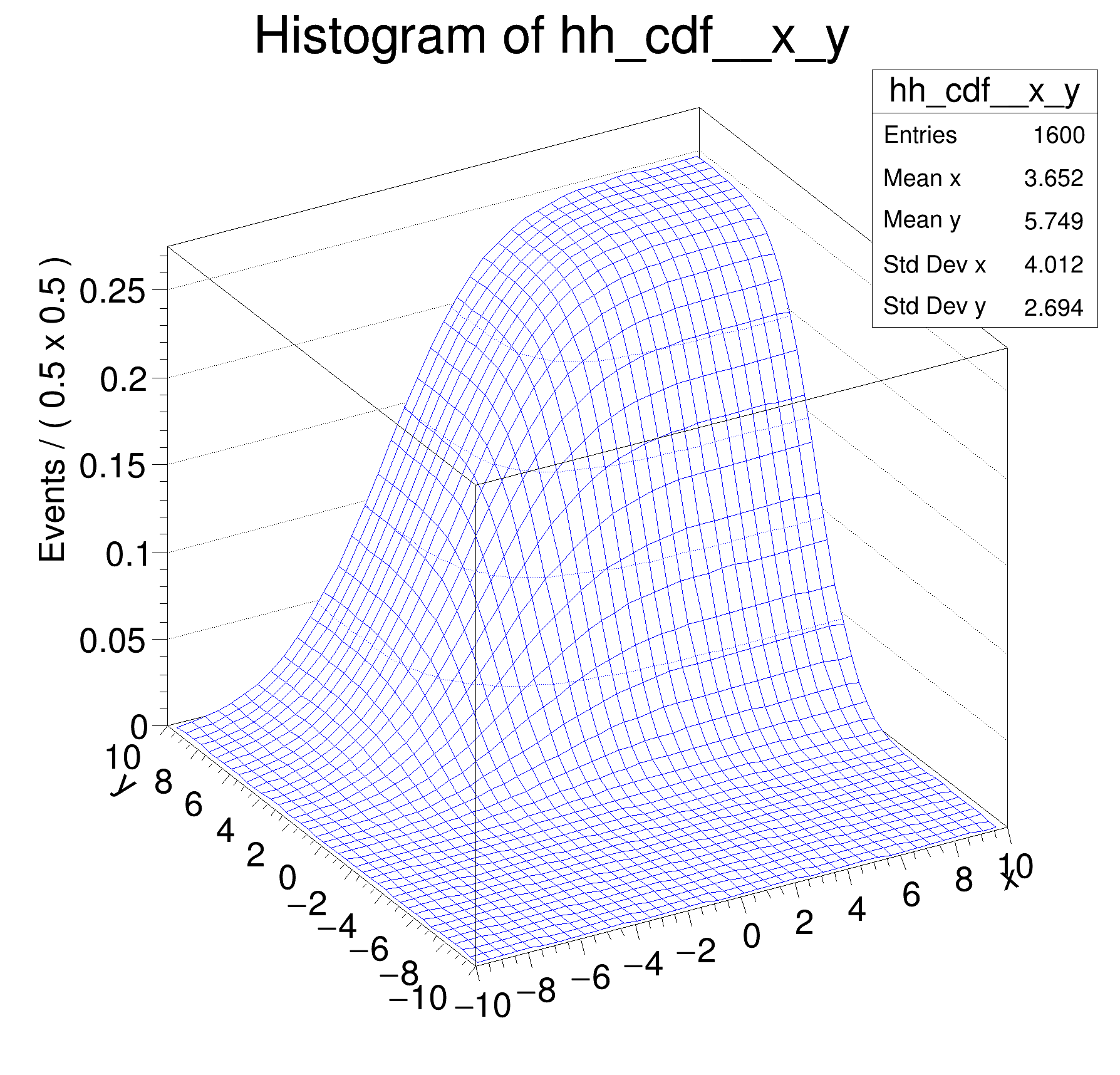ROOT   Reference Guiderf308_normintegration2d.C File Reference

## Detailed Description

Multidimensional models: normalization and integration of p.d.fs, construction of cumulative distribution functions from p.d.f.s in two dimensions␛[1mRooFit v3.60 -- Developed by Wouter Verkerke and David Kirkby␛[0m
Copyright (C) 2000-2013 NIKHEF, University of California & Stanford University
gxy = 0.485672
gx_Norm[x,y] = 0.0129332
gx_Int[x,y] = 37.5523
gx_Norm[x] = 0.106896
gx_Norm[y] = 0.120989
[#1] INFO:Eval -- RooRealVar::setRange(x) new range named 'signal' created with bounds [-5,5]
[#1] INFO:Eval -- RooRealVar::setRange(y) new range named 'signal' created with bounds [-3,3]
gx_Int[x,y|signal]_Norm[x,y] = 0.572035
[#0] WARNING:InputArguments -- RooAbsReal::createHistogram(gxy_cdf_Int[x_prime,y_prime|CDF]_Norm[x_prime,y_prime]) WARNING extended mode requested for a non-pdf object, ignored
#include "RooRealVar.h"
#include "RooGaussian.h"
#include "RooConstVar.h"
#include "RooProdPdf.h"
#include "RooAbsReal.h"
#include "RooPlot.h"
#include "TCanvas.h"
#include "TAxis.h"
#include "TH1.h"
using namespace RooFit;
{
// S e t u p m o d e l
// ---------------------
// Create observables x,y
RooRealVar x("x", "x", -10, 10);
RooRealVar y("y", "y", -10, 10);
// Create p.d.f. gaussx(x,-2,3), gaussy(y,2,2)
RooGaussian gx("gx", "gx", x, RooConst(-2), RooConst(3));
RooGaussian gy("gy", "gy", y, RooConst(+2), RooConst(2));
// Create gxy = gx(x)*gy(y)
RooProdPdf gxy("gxy", "gxy", RooArgSet(gx, gy));
// R e t r i e v e r a w & n o r m a l i z e d v a l u e s o f R o o F i t p . d . f . s
// --------------------------------------------------------------------------------------------------
// Return 'raw' unnormalized value of gx
cout << "gxy = " << gxy.getVal() << endl;
// Return value of gxy normalized over x _and_ y in range [-10,10]
RooArgSet nset_xy(x, y);
cout << "gx_Norm[x,y] = " << gxy.getVal(&nset_xy) << endl;
// Create object representing integral over gx
// which is used to calculate gx_Norm[x,y] == gx / gx_Int[x,y]
cout << "gx_Int[x,y] = " << igxy->getVal() << endl;
// NB: it is also possible to do the following
// Return value of gxy normalized over x in range [-10,10] (i.e. treating y as parameter)
RooArgSet nset_x(x);
cout << "gx_Norm[x] = " << gxy.getVal(&nset_x) << endl;
// Return value of gxy normalized over y in range [-10,10] (i.e. treating x as parameter)
RooArgSet nset_y(y);
cout << "gx_Norm[y] = " << gxy.getVal(&nset_y) << endl;
// I n t e g r a t e n o r m a l i z e d p d f o v e r s u b r a n g e
// ----------------------------------------------------------------------------
// Define a range named "signal" in x from -5,5
x.setRange("signal", -5, 5);
y.setRange("signal", -3, 3);
// Create an integral of gxy_Norm[x,y] over x and y in range "signal"
// This is the fraction of of p.d.f. gxy_Norm[x,y] which is in the
// range named "signal"
RooAbsReal *igxy_sig = gxy.createIntegral(RooArgSet(x, y), NormSet(RooArgSet(x, y)), Range("signal"));
cout << "gx_Int[x,y|signal]_Norm[x,y] = " << igxy_sig->getVal() << endl;
// C o n s t r u c t c u m u l a t i v e d i s t r i b u t i o n f u n c t i o n f r o m p d f
// -----------------------------------------------------------------------------------------------------
// Create the cumulative distribution function of gx
// i.e. calculate Int[-10,x] gx(x') dx'
RooAbsReal *gxy_cdf = gxy.createCdf(RooArgSet(x, y));
// Plot cdf of gx versus x
TH1 *hh_cdf = gxy_cdf->createHistogram("hh_cdf", x, Binning(40), YVar(y, Binning(40)));
hh_cdf->SetLineColor(kBlue);
new TCanvas("rf308_normintegration2d", "rf308_normintegration2d", 600, 600);
hh_cdf->GetZaxis()->SetTitleOffset(1.8);
hh_cdf->Draw("surf");
}
@ kBlue
Definition: Rtypes.h:64
RooAbsReal is the common abstract base class for objects that represent a real value and implements f...
Definition: RooAbsReal.h:60
TH1 * createHistogram(const char *varNameList, Int_t xbins=0, Int_t ybins=0, Int_t zbins=0) const
Create and fill a ROOT histogram TH1, TH2 or TH3 with the values of this function for the variables w...
Double_t getVal(const RooArgSet *normalisationSet=nullptr) const
Evaluate object.
Definition: RooAbsReal.h:90
RooAbsReal * createIntegral(const RooArgSet &iset, const RooCmdArg &arg1, const RooCmdArg &arg2=RooCmdArg::none(), const RooCmdArg &arg3=RooCmdArg::none(), const RooCmdArg &arg4=RooCmdArg::none(), const RooCmdArg &arg5=RooCmdArg::none(), const RooCmdArg &arg6=RooCmdArg::none(), const RooCmdArg &arg7=RooCmdArg::none(), const RooCmdArg &arg8=RooCmdArg::none()) const
Create an object that represents the integral of the function over one or more observables listed in ...
Definition: RooAbsReal.cxx:560
RooArgSet is a container object that can hold multiple RooAbsArg objects.
Definition: RooArgSet.h:28
Plain Gaussian p.d.f.
Definition: RooGaussian.h:25
RooProdPdf is an efficient implementation of a product of PDFs of the form.
Definition: RooProdPdf.h:31
RooRealVar represents a variable that can be changed from the outside.
Definition: RooRealVar.h:35
virtual void SetTitleOffset(Float_t offset=1)
Set distance between the axis and the axis title.
Definition: TAttAxis.cxx:294
virtual void SetLineColor(Color_t lcolor)
Set the line color.
Definition: TAttLine.h:40
The Canvas class.
Definition: TCanvas.h:27
The TH1 histogram class.
Definition: TH1.h:56
TAxis * GetZaxis()
Definition: TH1.h:318
virtual void Draw(Option_t *option="")
Draw this histogram with options.
Definition: TH1.cxx:2998
RooCmdArg NormSet(const RooArgSet &nset)
RooCmdArg YVar(const RooAbsRealLValue &var, const RooCmdArg &arg=RooCmdArg::none())
RooConstVar & RooConst(Double_t val)
RooCmdArg Binning(const RooAbsBinning &binning)
Double_t y[n]
Definition: legend1.C:17
Double_t x[n]
Definition: legend1.C:17
The namespace RooFit contains mostly switches that change the behaviour of functions of PDFs (or othe...
Ta Range(0, 0, 1, 1)
Date
07/2008

Definition in file rf308_normintegration2d.C.